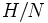# Group of prime power order having a larger abelianization than any proper subgroup has class two

## Statement

Let$P$ be a group of prime power order with the property that the order of the abelianization of$P$ (i.e., the quotient of$P$ by its commutator subgroup) is larger than that of any proper subgroup of$P$. Then,$P$ is a Group of nilpotency class two (?).

## Proof

We prove this for any prime$p$ by assuming$P$ to be a minimal counterexample for that prime$p$. In other words, we show that if$P$ is a finite$p$-group having a larger abelianization than every proper subgroup but does not have class two, we have a contradiction.

1. Let$N$ be a minimal normal subgroup of$P$ contained in$P' = [P,P]$: Note that such a$N$ exists because$[P,P]$ is a nontrivial normal subgroup of$P$.
2. Let$H$ be a proper subgroup of$P$ containing$N$. Then, the abelianization of$P/N$ is larger in size than the abelianization of$H/N$: We have$[(P/N):(P/N)'] = [P:P']$ because$N \le P'$ (using fact (1)).$[P:P'] > [H:H']$ by assumption. Finally,$[H:H'] \ge [(H/N):(H/N)']$ (equality need not hold since$N$ is not necessarily contained in$H'$. Combining all these,$[(P/N):(P/N)'] > [(H/N):(H/N)']$.
3.$N = [[P,P],P]$: From step (2), we know that$P/N$ has a larger abelianization than any proper subgroup. By the minimal counterexample nature of$P$,$P/N$ must have class two. Thus,$[[P,P],P] \le N$. But$[[P,P],P]$ is a normal subgroup of$P$ and$N$ is minimal normal. Moreover,$P$ has class greater than two, so$[[P,P],P]$ is nontrivial. This forces$N = [[P,P],P]$.
4. Let$C = C_P(P')$ and$D = P'/(P' \cap Z(P))$. Then, there is a map$(G/C) \times D \to N$ given by$(gC,x) \mapsto [g,x]$, and this map is homomorphic in each argument: Using fact (2), we see that the map is well-defined, and homomorphic in both arguments.